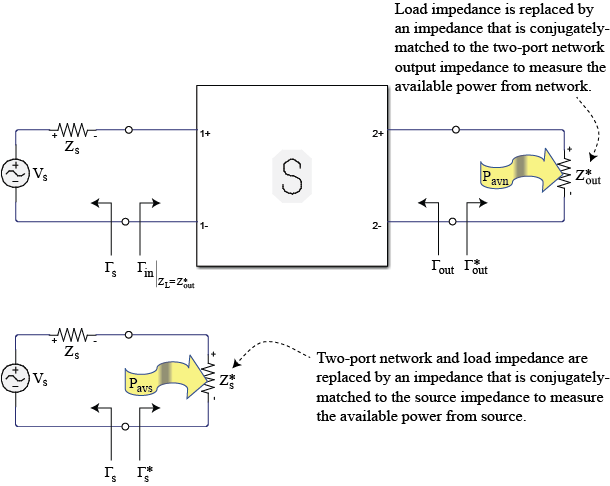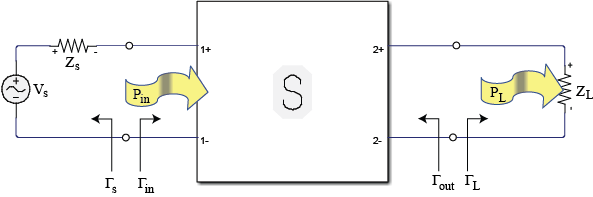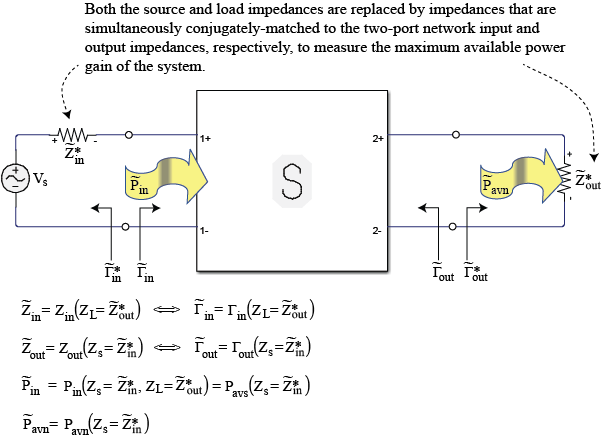# powergain

Calculate power gain from two-port S-parameters

## Syntax

``g = powergain(s_params,z0,zs,zl,'Gt')``
``g = powergain(s_params,z0,zs,'Ga')``
``g = powergain(s_params,z0,zl,'Gp')``
``g = powergain(s_params,'Gmag')``
``g = powergain(s_params,'Gmsg')``
``g = powergain(hs,zs,zl,'Gt')``
``g = powergain(hs,zs,'Ga')``
``g = powergain(hs,zl,'Gp')``
``g = powergain(hs,'Gmag')``
``g = powergain(hs,'Gmsg')``

## Description

````g = powergain(s_params,z0,zs,zl,'Gt')` calculates the transducer power gain of the 2-port network by: ${G}_{t}=\frac{{P}_{L}}{{P}_{\text{avs}}}=\frac{\left(1-{|{\Gamma }_{S}|}^{2}\right){|{S}_{21}|}^{2}\left(1-{|{\Gamma }_{L}|}^{2}\right)}{{|\left(1-{S}_{11}{\Gamma }_{S}\right)\left(1-{S}_{22}{\Gamma }_{L}\right)-{S}_{12}{S}_{21}{\Gamma }_{S}{\Gamma }_{L}|}^{2}}$where, PL is the output power and Pavs is the maximum input power.ΓL and ΓS are the reflection coefficients defined as:$\begin{array}{l}{\Gamma }_{S}=\frac{{Z}_{S}-{Z}_{0}}{{Z}_{S}+{Z}_{0}}\\ {\Gamma }_{L}=\frac{{Z}_{L}-{Z}_{0}}{{Z}_{L}+{Z}_{0}}\end{array}$ ```
````g = powergain(s_params,z0,zs,'Ga')` calculates the available power gain of the 2-port network by: ${G}_{a}=\frac{{P}_{\text{avn}}}{{P}_{\text{avs}}}=\frac{\left(1-{|{\Gamma }_{S}|}^{2}\right){|{S}_{21}|}^{2}}{{|1-{S}_{11}{\Gamma }_{S}|}^{2}\left(1-{|{\Gamma }_{out}|}^{2}\right)}$where Pavn is the available output power from the network.Γout is given by:${\Gamma }_{\text{out}}={S}_{22}+\frac{{S}_{12}{S}_{21}{\Gamma }_{S}}{1-{S}_{11}{\Gamma }_{S}}$ ```
````g = powergain(s_params,z0,zl,'Gp')` calculates the operating power gain of the 2-port network by: ${G}_{p}=\frac{{P}_{L}}{{P}_{\text{in}}}=\frac{{|{S}_{21}|}^{2}\left(1-{|{\Gamma }_{L}|}^{2}\right)}{\left(1-{|{\Gamma }_{\text{in}}|}^{2}\right){|1-{S}_{22}{\Gamma }_{L}|}^{2}}$where Pin is the input power.Γin is given by:${\Gamma }_{\text{in}}={S}_{11}+\frac{{S}_{12}{S}_{21}{\Gamma }_{L}}{1-{S}_{22}{\Gamma }_{L}}$ ```
````g = powergain(s_params,'Gmag')` calculates the maximum available power gain of the 2-port network. by: ${G}_{\text{mag}}=\frac{|{S}_{21}|}{|{S}_{12}|}\left(K-\sqrt{{K}^{2}-1}\right)$where K is the stability factor.```

example

````g = powergain(s_params,'Gmsg')` calculates the maximum stable gain of the 2-port network by:${G}_{msg}=\frac{|{S}_{21}|}{|{S}_{12}|}$```
````g = powergain(hs,zs,zl,'Gt')` calculates the transducer power gain of the network represented by the S-parameter object `hs`.```
````g = powergain(hs,zs,'Ga')` calculates the available power gain of the network represented by the S-parameter object `hs`.```
````g = powergain(hs,zl,'Gp')` calculates the operating power gain of the network represented by the S-parameter object `hs`.```
````g = powergain(hs,'Gmag')` calculates the maximum available power gain of the network represented by the S-parameter object `hs`.```
````g = powergain(hs,'Gmsg')` calculates the maximum stable gain of the network represented by the S-parameter object `hs`.```

## Examples

collapse all

Calculate power gains for a sample 2-port network.

```s11 = 0.61*exp(1j*165/180*pi); s21 = 3.72*exp(1j*59/180*pi); s12 = 0.05*exp(1j*42/180*pi); s22 = 0.45*exp(1j*(-48/180)*pi); sparam = [s11 s12; s21 s22]; z0 = 50; zs = 10 + 1j*20; zl = 30 - 1j*40;```

Calculate the transducer power gain of the network

`Gt = powergain(sparam,z0,zs,zl,'Gt')`
```Gt = 4.7066 ```

Calculate the available power gain of the network

`Ga = powergain(sparam,z0,zs,'Ga')`
```Ga = 11.4361 ```

Note that, as expected, the available power gain is larger than the transducer power gain, `Gt`. The two become identical when `Gt` is measured with a matched load impedance:

```zl_matched = gamma2z(gammaout(sparam, z0, zs)', z0); Gt_zl_matched = powergain(sparam, z0, zs, zl_matched, 'Gt')```
```Gt_zl_matched = 11.4361 ```

Calculate the operating power gain of the network

`Gp = powergain(sparam,z0,zl,'Gp')`
```Gp = 10.5098 ```

Note that, as expected, the operating power gain is larger than the transducer power gain, `Gt`. The two become identical when `Gt` is measured with a matched source impedance:

```zs_matched = gamma2z(gammain(sparam, z0, zl)', z0); Gt_zs_matched = powergain(sparam, z0, zs_matched, zl, 'Gt')```
```Gt_zs_matched = 10.5098 ```

Calculate the maximum available power gain of the network

`Gmag = powergain(sparam,'Gmag')`
```Gmag = 41.5032 ```

Note that, as expected, the maximum available power gain is larger than the available power gain `Ga`, the transducer power gain, `Gt`, and the operating power gain, `Gp`. They all become identical when measured with simultaneously matched source and load impedances:

```zs_matched_sim = gamma2z(gammams(sparam), z0); zl_matched_sim = gamma2z(gammaout(sparam, z0, zs_matched_sim)', z0)```
```zl_matched_sim = 33.6758 + 91.4816i ```

That impedance can be also obtained directly using:

`zl_matched_sim = gamma2z(gammaml(sparam), z0)`
```zl_matched_sim = 33.6758 + 91.4816i ```
`Ga_matched_sim = powergain(sparam, z0, zs_matched_sim, 'Ga')`
```Ga_matched_sim = 41.5032 ```
`Gt_matched_sim = powergain(sparam, z0, zs_matched_sim, zl_matched_sim, 'Gt')`
```Gt_matched_sim = 41.5032 ```
`Gp_matched_sim = powergain(sparam, z0, zl_matched_sim, 'Gp')`
```Gp_matched_sim = 41.5032 ```

When the scattering parameters represent a network that is not unconditionally stable, there is no set of source and load impedances that provide simultaneous matching. In this case, the maximum available power is infinite, but truly meaningless because the network is unstable.

To make the previously defined network conditionally stable, it is enough to increase the magnitude of the backward propagation scattering parameter, `s12`:

```s12_cond_stable = 0.06*exp(1j*42/180*pi); sparam_cond_stable = [s11 s12_cond_stable; s21 s22];```

To verify that the network is conditionally stable, check that the stability factor, K, is smaller than 1:

`K = stabilityk(sparam_cond_stable)`
```K = 0.9695 ```

An attempt to calculate the maximum available gain of the network yields a NaN:

`Gmag_cond_stable = powergain(sparam_cond_stable,'Gmag')`
```Gmag_cond_stable = NaN ```

Instead, the maximum stable gain, ${\mathit{G}}_{\mathrm{msg}}$, should be used.

Calculate the maximum stable power gain of the network

`Gmsg_cond_stable = powergain(sparam_cond_stable,'Gmsg')`
```Gmsg_cond_stable = 62.0000 ```

The maximum stable power gain is only meaningful when the network is not unconditionally stable.

## Input Arguments

collapse all

2-port S-parameters, specified as an RF Toolbox™ S-parameter object.

2-port S-parameters, specified as a complex 2-by-2-by-N array.

Reference impedance in ohms, specified as a positive scalar. If the first input argument is an S-parameter object `hs`, the function uses `hs.Impedance` for the reference impedance.

Load impedance in ohms, specified as a positive scalar.

Source impedance in ohms, specified as a positive scalar.

## Output Arguments

collapse all

Unit less power gain values, returned as a vector. To obtain power gain in decibels, use `10*log10(g)`.

If the specified type of power gain is undefined for one or more of the specified S-parameter values in `s_params`, the `powergain` function returns `NaN`. As a result, `g` is either `NaN` or a vector that contains one or more `NaN` entries.

collapse all

### Transducer Power Gain

Gt = PL/Pavs is the ratio of power delivered to the load to the power available from the source. This depends on both `ZS` and `ZL`.### Available Power Gain

Ga = Pavn/Pavs is the ratio of the power available from the two-port network to the power available from the source. Available gain is the transducer power gain when load impedance is equal to output impedance. Thus Ga depends only on Zs.### Operating Power Gain

Gp = PL/Pin is the ratio of power dissipated in the load `Z`L to the power delivered to the input of the two-port network. This gain is independent of `Z`S, although some active circuits are strongly dependent on the input matching conditions.### Maximum Available Power Gain and Maximum Stable Power Gain

Maximum available power gain, Gmag is Gawith matched input that is `Z`S is equal to `Z`in

In the case of conditionally stable two-port networks (K<1) where the maximum available power gain result is meaningless, the maximum stable power gain, Gmsg, should be used.